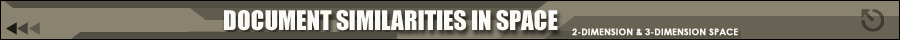## Introduction of the Project

This project, as a part of the Information Storage and Retrieval course is about the visualization of document similarity. We show the document space that represents the similarity / dissimilarity between the documents. In our project, we also consider query as a document and therefore represent it in the document space. For the purpose of calculating the distance between the documents and query, we have used Euclidean distance and Cosine Measure. Along with these distance measure methods, the FastMap algorithm is used to map the position of documents and query. The visualization is represented in 2-D and 3-D graphics therefore it is possible to compare how the document space features are represented differently.

Document space is the representation of the set of documents. There can be different ways of representing documents, however mostly it is used to show the distance or the similarity between different documents. When documents are close each other in the document space this represents that they are similar or relevant document in the set. When documents are far apart each other in the document space this means they are not similar or relevant each other. Therefore, to represent documents in document space, the distances between documents are necessary.

Document distances can be calculated with several distance measurement methods. Mainly used methods are vector-based such as Euclidean measure and Cosine measure. In vector-based models, documents and query are represented with their weights which correspond to the importance of the term in the document. Here in our project, term weights are related to the frequency of the terms in the document. Each terms in the document will have a vector value, by which the distace between documents will be calculated. The vector is calculated as following formula for the first term in a document, for example.

 Vector = ( term 1 frequency )/sqrt[( term 1 freq )2+( term 2 freq )2+......+( term n freq )2]

We used Euclidean Distance Measure for calculating the document distances between two documents or between document and query. Euclidean distance is calculated with the formula below.

 E.D(D,Q) = sqrt[ sigma( tn - qn )2 ]

Created By: Shruti Parikh, Sueyeon Syn, Kittipong Techapanichgul, Zhiwen Yu

December 16, 2004